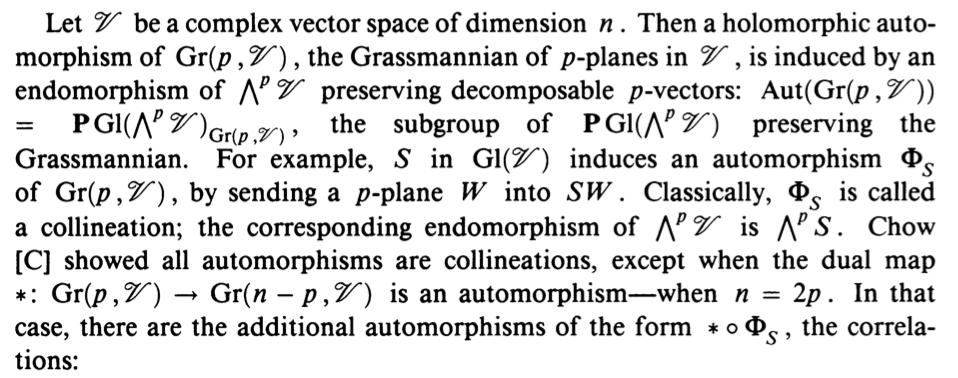# What are the automorphisms of a Grassmannian?

I want to know what are the holomorphic automorphisms of a Grassmannian. Can someone tell me this?

• ams.org/journals/proc/1989-106-01/S0002-9939-1989-0938909-8 Jul 1, 2014 at 6:29
• @Carlo: I suggest that you promote your comment to an answer. Jul 1, 2014 at 8:17
• It probably would have been a good idea to quote the paper by Wei-Liang Chow, On the geometry of algebraic homogeneous spaces, Ann. of Math. 50 (1949), 32–67, since that is where the original argument is made. Jul 1, 2014 at 16:17• Demazure also gave a complete characterization of automorphism groups of generalized Grassmannians $G/P$, "Automorphismes et deformations des varietes de Borel", Invent. Math. 1977. Essentially, the automorphism group of $G/P$ is generated by $G$ (adjoint form) together with certain automorphisms of the Dynkin diagram. The above is a special case, since $Gr(k,n) = PGL_n/P$. Jul 1, 2014 at 14:02